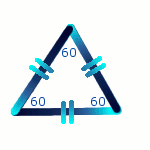# Angle Rules

## Complementary & Supplementary Angles

 NAME RULE DIAGRAM Complementary angles Add to 90 degreesSupplementary angles Add to 180 degrees(Hint to remember these: C in the alphabet and 90 when counting both come before S and 180.)

## Vertically Opposite Angles

 NAME RULE DIAGRAM Vertically opposite angles Equal## Angles of Parallel Lines

 NAME RULE DIAGRAM Corresponding angles (F shape)Co-interior angles Add to 180 degrees (U shape)Alternate angles Equal (Z shape)## Angles of Triangles

 NAME RULE DIAGRAM Angle sum of triangle Add to 180 degreesEquilateral triangle All angles equal 60 degreesIsosceles triangle Base angles are equalExterior angle of triangle The exterior angle equals thesum of the two interior opposite angles.## Question - Angle Sum of Triangle

To show that the angle sum of a triangle equals 180 degrees, draw a triangle, tear the angles and rearrange them into a straight line. Remember that the number of degrees in a straight line is 180 degrees.

Do a similar activity to show that the angles of a quadrilateral add to 360 degrees.

## Angles of Quadrilaterals

 NAME RULE DIAGRAM Angle sum of quadrilateral Add to 360 degreesOpposite angles of parallelogram Equal## Angles of Circles

 NAME RULE DIAGRAM Radius meets tangent The radius meets the tangent at right angles.Angle at centre The angle subtended at the centre of the circle is twice the angle at the circumference.(arrow shape)Angle at circumference Angles subtended by the same chord are equal.(butterfly shape)Opposite angles of cyclic quadrilateral EqualExterior angle of cyclic quadrilateral The exterior angle of a cyclic quadrilateral is equal to the interior opposite angle.